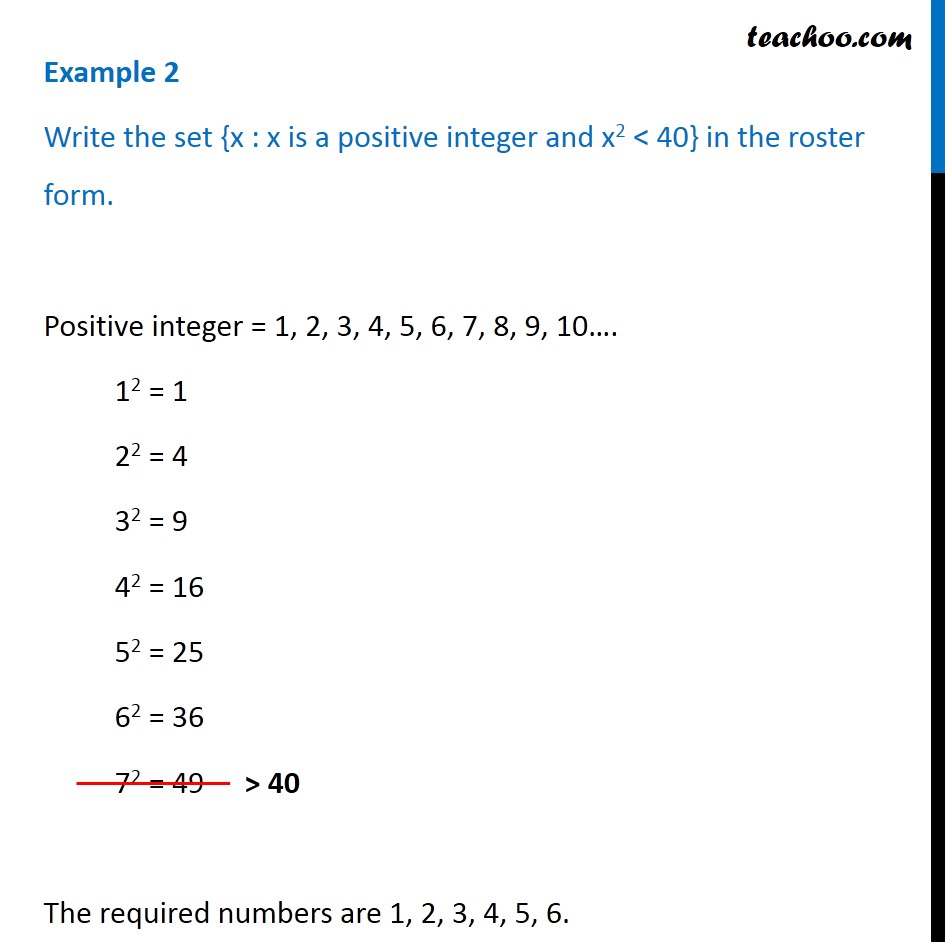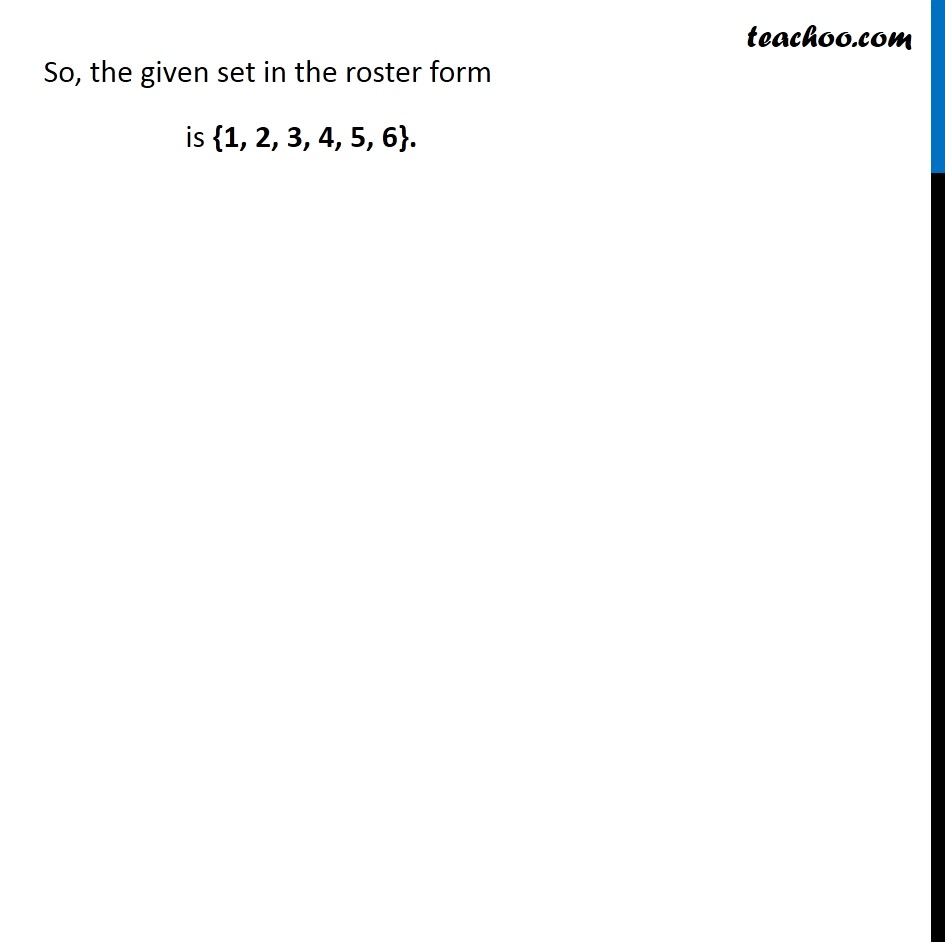1. Chapter 1 Class 11 Sets
2. Concept wise
3. Depicition of sets - Roster form

Transcript

Example 2 Write the set {x : x is a positive integer and x2 < 40} in the roster form. Positive integer = 1, 2, 3, 4, 5, 6, 7, 8, 9, 10…. 12 = 1 22 = 4 32 = 9 42 = 16 52 = 25 62 = 36 72 = 49 The required numbers are 1, 2, 3, 4, 5, 6. So, the given set in the roster form is {1, 2, 3, 4, 5, 6}.

Depicition of sets - Roster form